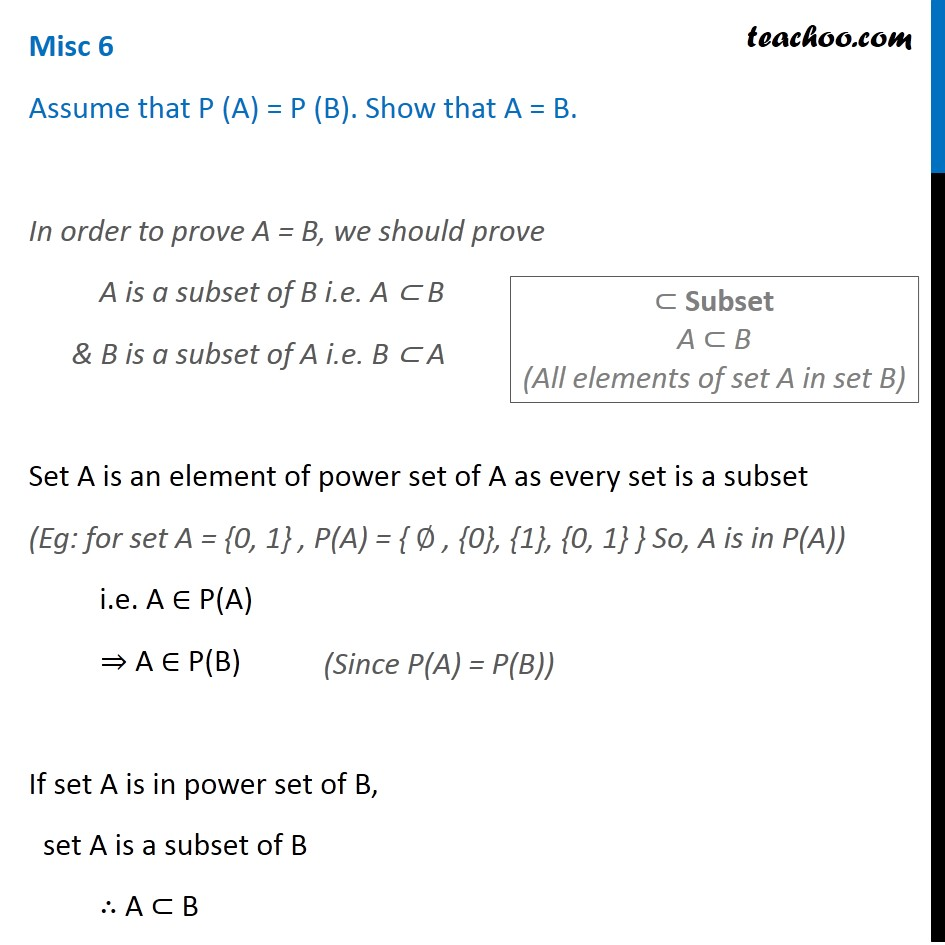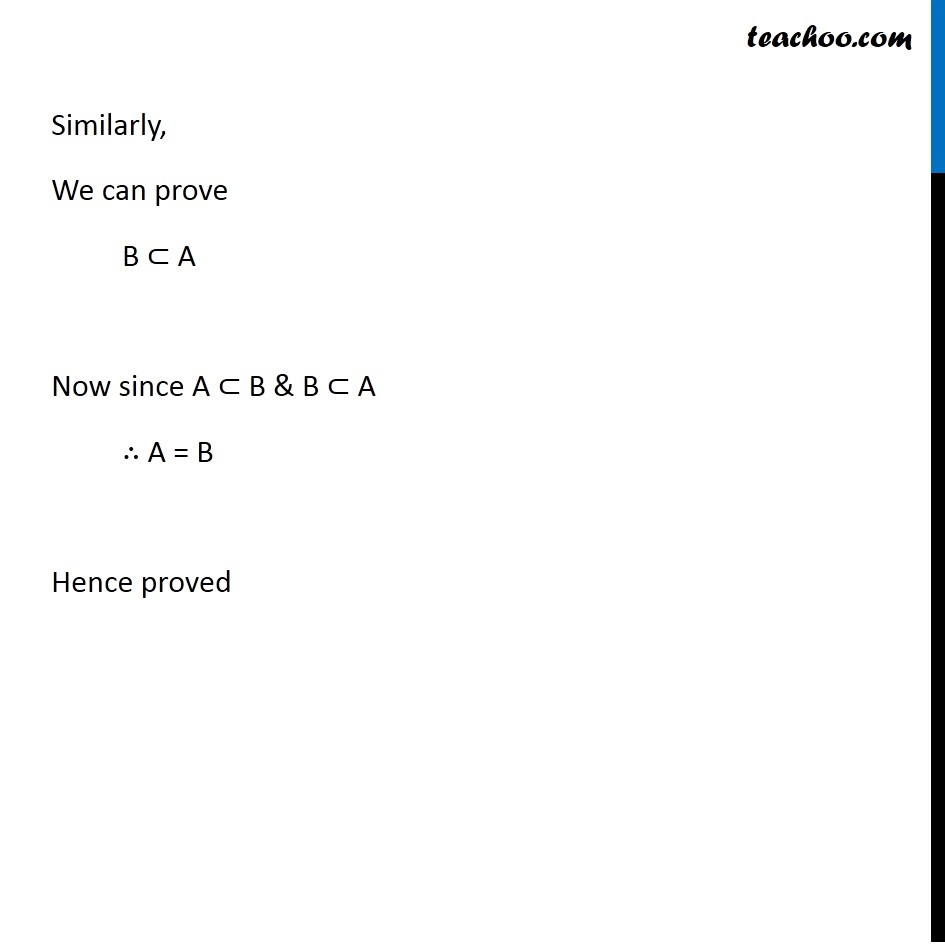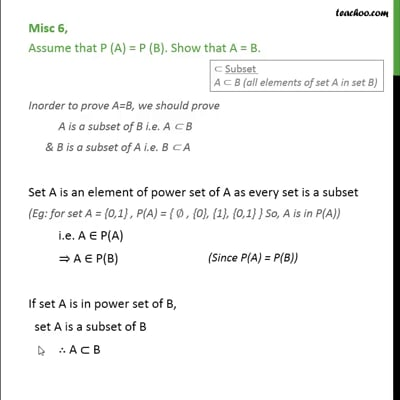Miscellaneous

Chapter 1 Class 11 Sets
Serial order wiseThis video is only available for Teachoo black users

Solve all your doubts with Teachoo Black (new monthly pack available now!)

### Transcript

Misc 6 Assume that P (A) = P (B). Show that A = B. In order to prove A = B, we should prove A is a subset of B i.e. A ⊂ B & B is a subset of A i.e. B ⊂ A Set A is an element of power set of A as every set is a subset (Eg: for set A = {0, 1} , P(A) = { ∅ , {0}, {1}, {0, 1} } So, A is in P(A)) i.e. A ∈ P(A) ⇒ A ∈ P(B) If set A is in power set of B, set A is a subset of B ∴ A ⊂ B ⊂ Subset A ⊂ B (All elements of set A in set B) Similarly, We can prove B ⊂ A Now since A ⊂ B & B ⊂ A ∴ A = B Hence proved